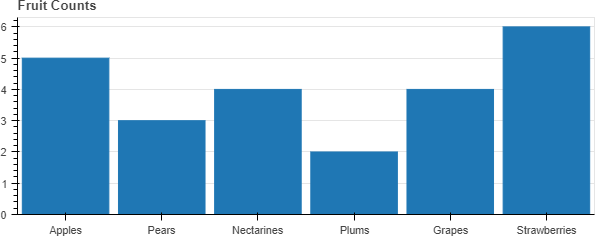# How to set an initial zoom on a FactorRange

For example, suppose I have the following bar plot from the documentation:

from bokeh.io import show, output_notebook
from bokeh.plotting import figure

output_notebook()

# Here is a list of categorical values (or factors)

fruits = [‘Apples’, ‘Pears’, ‘Nectarines’, ‘Plums’, ‘Grapes’, ‘Strawberries’]

# Set the x_range to the list of categories above

p = figure(x_range=fruits, plot_height=250, title=“Fruit Counts”)

# Categorical values can also be used as coordinates

p.vbar(x=fruits, top=[5, 3, 4, 2, 4, 6], width=0.9)

# Set some properties to make the plot look better

p.xgrid.grid_line_color = None
p.y_range.start = 0

show§

``How do I set it so that the initial zoom shows only the first four categories (but the user can pan around to see the other two, zoom out, etc.)?

Hi,

There is not currently any way to do this, plots with FactorRange on an axis will always default to showing all the factors. Depending on your use case, an alternative might be to use a "fake" categorical axis, i.e. use a basic numeric Range1d with numbeic data, but then use a ticker and tick formatter show string labels for specific locations.

Thanks,

Bryan

···

On May 30, 2018, at 11:39, [email protected] wrote:

For example, suppose I have the following bar plot from the documentation:

from bokeh.io import show, output_notebook
from bokeh.plotting import figure

output_notebook()

# Here is a list of categorical values (or factors)
fruits = ['Apples', 'Pears', 'Nectarines', 'Plums', 'Grapes', 'Strawberries']

# Set the x_range to the list of categories above
p = figure(x_range=fruits, plot_height=250, title="Fruit Counts")

# Categorical values can also be used as coordinates
p.vbar(x=fruits, top=[5, 3, 4, 2, 4, 6], width=0.9)

# Set some properties to make the plot look better
p.xgrid.grid_line_color = None
p.y_range.start = 0

show(p)

How do I set it so that the initial zoom shows only the first four categories (but the user can pan around to see the other two, zoom out, etc.)?

--
You received this message because you are subscribed to the Google Groups "Bokeh Discussion - Public" group.
To unsubscribe from this group and stop receiving emails from it, send an email to [email protected].
To post to this group, send email to [email protected].
To view this discussion on the web visit https://groups.google.com/a/continuum.io/d/msgid/bokeh/641ff55f-16a7-4d93-8acc-ffd2b52aa4dc%40continuum.io.

Understood. Thanks!

···

On Wednesday, May 30, 2018 at 11:56:07 AM UTC-7, Bryan Van de ven wrote:

Hi,

There is not currently any way to do this, plots with FactorRange on an axis will always default to showing all the factors. Depending on your use case, an alternative might be to use a “fake” categorical axis, i.e. use a basic numeric Range1d with numbeic data, but then use a ticker and tick formatter show string labels for specific locations.

Thanks,

Bryan

On May 30, 2018, at 11:39, [email protected] wrote:

For example, suppose I have the following bar plot from the documentation:

from bokeh.io import show, output_notebook

from bokeh.plotting import figure

output_notebook()

# Here is a list of categorical values (or factors)

fruits = [‘Apples’, ‘Pears’, ‘Nectarines’, ‘Plums’, ‘Grapes’, ‘Strawberries’]

# Set the x_range to the list of categories above

p = figure(x_range=fruits, plot_height=250, title=“Fruit Counts”)

# Categorical values can also be used as coordinates

p.vbar(x=fruits, top=[5, 3, 4, 2, 4, 6], width=0.9)

# Set some properties to make the plot look better

p.xgrid.grid_line_color = None

p.y_range.start = 0

show§

How do I set it so that the initial zoom shows only the first four categories (but the user can pan around to see the other two, zoom out, etc.)?

You received this message because you are subscribed to the Google Groups “Bokeh Discussion - Public” group.

To unsubscribe from this group and stop receiving emails from it, send an email to [email protected].

To post to this group, send email to [email protected].

To view this discussion on the web visit https://groups.google.com/a/continuum.io/d/msgid/bokeh/641ff55f-16a7-4d93-8acc-ffd2b52aa4dc%40continuum.io.